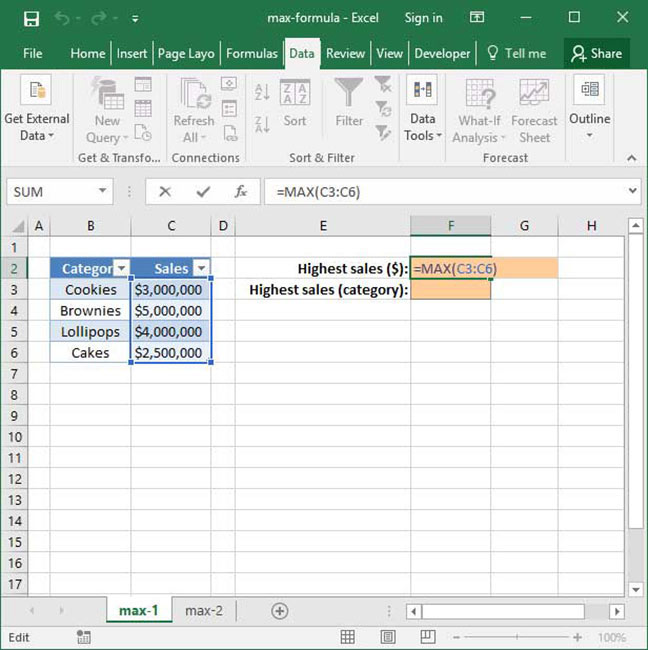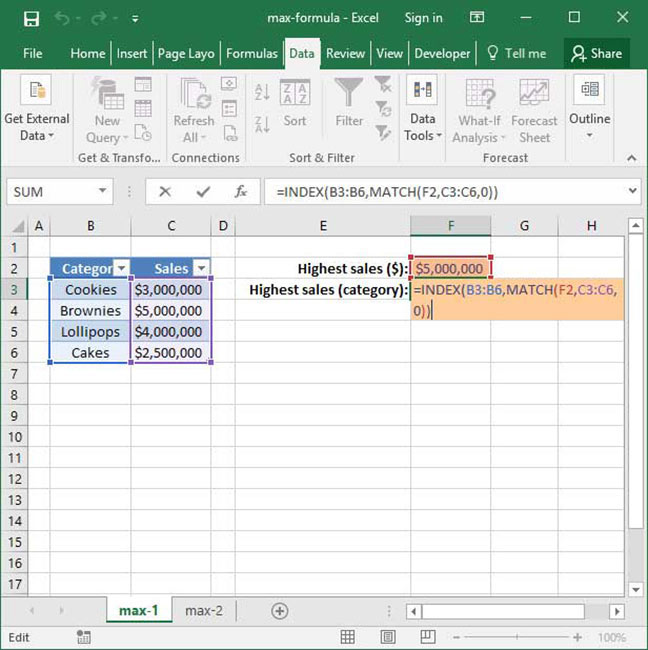# Using Excel's MAX Formula

Oftentimes, you'll want to use Excel to find the maximum value in a range or series of numbers. For example, given a list of salespeople and corresponding dollar sales in any given month, you might want to find the maximum dollar sales to identify the highest-performing salesperson.

One way to accomplish this is by looking through your list of values and manually identifying the largest one. But that seems particularly time consuming and error-prone — especially if you're working with a big list. That's why Excel includes the MAX function. Using MAX, you can easily construct formulas that identify the largest value in a set. Keep reading to find out how.

## A simple use of the MAX function

The MAX function is very simple to use; its basic syntax is as follows:

`=MAX(number_or_range_1, number_or_range_2 (optional)...)`

Note a couple of things here: first of all, the number_or_range arguments can contain either a number, like 4, or a range, like B4:B6. Second, you can insert as many number_or_range arguments as you want into the formula. Given these, Excel will output the largest value contained in either the numbers or ranges specified.

Let's take a look at a simple example to demonstrate this formula in action:

`=MAX(4, 5, 6)Output: 6`

The above formula outputs the value 6, because 6 is the highest value supplied as an argument to the function.

Here's another example using a larger series of numbers:

`=MAX(-7, 15, -100, 0, 3)Output: 15`

This formula outputs the value 15, because 15 is the highest value supplied to the function.

## Using MAX with a range

Per the above, the MAX function can also be used with a range of values rather than a series of discrete inputs. Take a look at the following example, in which we use MAX to calculate the highest dollar sales by product category:`=MAX(C3:C6)Output: \$5,000,000`

In the above example, we use MAX on the range of values representing dollar sales by category to extract the highest value — \$5,000,000.

This is certainly useful, but what we really care about here is the name of the best-performing category — not necessarily the dollar value of sales within that category. Is there a way to extract just the name of the best-performing category using the MAX function?

The answer is yes — but to do so, we'll need to use a much more complicated function called INDEX MATCH. Take a look at the below example, in which we've combined MAX with INDEX MATCH to identify the best-performing category of the month:`=INDEX(B3:B6, MATCH(F2, C3:C6, 0))Output: "Brownies"`

This may seem complicated, but if you'd like to learn more, head over to our INDEX MATCH tutorial. Once you've reviewed it and have a handle on how to use INDEX MATCH, come back here and see whether you can figure out why the above formula works like it does.

## Other uses of MAX

Of course, MAX has many uses other than identifying the best-performing salespeople and categories. Here are some other ideas for how you can use MAX to make your life easier:

• Find the most recent day in a range of dates;
• Identify top-performing students by assignment;
• Find salespeople with the most sales in a given time range;
• Find the highest-recorded temperature in a given location; and
• Benchmark stock prices to maximum historical performance.